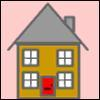#### You may also like### Odd Differences

The diagram illustrates the formula: 1 + 3 + 5 + ... + (2n - 1) = nÂ² Use the diagram to show that any odd number is the difference of two squares.### Iff### Smith and Jones

Mr Smith and Mr Jones are two maths teachers. By asking questions, the answers to which may be right or wrong, Mr Jones is able to find the number of the house Mr Smith lives in... Or not!

# Triangular Triples

##### Age 14 to 16Challenge Level

Chong Ching Tong from River Valley High School, Singapore and Andrei Lazanu, age12, School: No. 205 Bucharest, Romania approached this problem in different ways.

Here is Chong's working:

\begin{eqnarray} 8778 \times 2 = 17556\\ \sqrt{17556} \cong 132.5\\ \frac {(132 \times 133)}{2} = 8778\\ 10296 \times 2 = 20592\\ \sqrt{20592} \cong 143.5\\ \frac {(143 \times 144)}{2} = 10296 \\ 13530 \times 2 = 27060\\ \sqrt{27060} \cong 164.5\\ \frac {(164 \times 165)}{2} = 13530\\ (8778)^2 + (10296)^2 = (13530)^2 \end{eqnarray}

Here is Andrei's solution:

First I demonstrate that 8778, 10296 and 13530 are triangular numbers, i.e. they can be written in the form n(n+1)/2. In order to do this I decomposed the product of each of the three numbers by 2 in the hope to put it in the form n(n+1)/2. I found: $$8778\times2 = 2^2\times3\times 7\times 11\times 19 = 132\times133$$ So, $$8778 = \frac{132 \times 133}{2}$$ and so 8778 is a triangular number. $$10296\times 2 = 2^2\times 11\times13 = 143\times144$$ So, $$0296 = \frac{143 \times 144}{2}$$, and it is a triangular number. $$13530\times 2 = 2^2\times3\times5\times11\times41 = 164\times 165$$ So, $$3530 = \frac{164 \times 165}{2}$$ is also a triangular number.

Now, I demonstrate that the three numbers are a Pythagorean triple. The greatest number is 13530 $$13530^2= 183060900$$ $$8778^2+ 10296^2 = 77053284 + 106007616 = 183060900$$

So, the three numbers are a Pythagorean triple